# What is the Shape of a Pixel?

### Contents

#### The Shape of a Pixel

What is the shape of a pixel? At various times, I have a pixel as a square (often), a point (sometimes), or a rectangle (occasionally). I recall back in grad school doing some homework where we were treating pixels as hexagons.

As I haved worked through the last few posts on computing Feret diameters, though, I have started to entertain the possible usefulness of considering pixels to be circles. (See 29-Sep-2017, 24-Oct-2017, and 20-Feb-2018.) Let me try to explain why.

Here's a binary image with a single foreground blob (or "object," or "connected component.")

bw = imread('Martha''s Vineyard (30x20).png');
imshow(bw)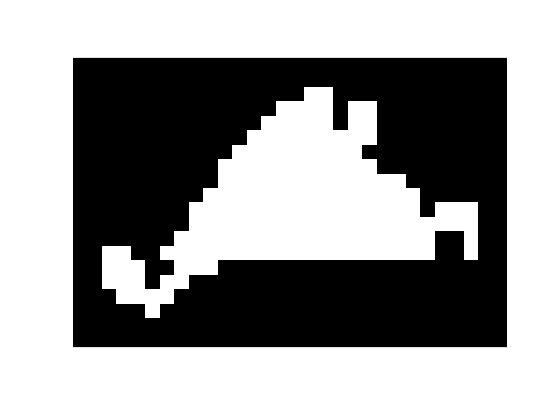Most of the time, we think of image pixels as being squares with unit area.

pixelgrid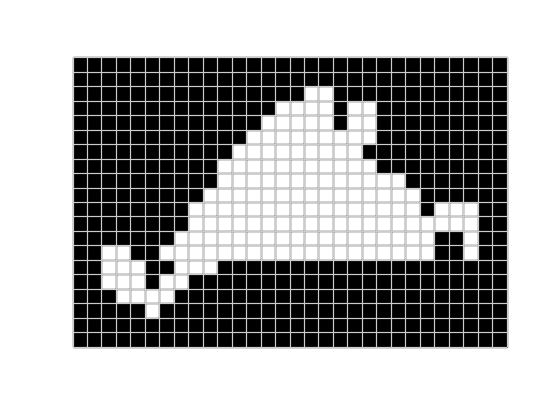We can use find to get the $x$- and $y$-coordinates of the pixel centers, and then we can use convhull to find their convex hull. As an optimization that I think will often reduce execution time and memory, I'm going to preprocess the input binary image here by calling bwperim. I'm not going to show that step everywhere in this example, though.

[y,x] = find(bwperim(bw));
hold on
plot(x,y,'.')
hold off
title('Pixel centers')
h = convhull(x,y);
x_hull = x(h);
y_hull = y(h);
hold on
hull_line = plot(x_hull,y_hull,'r*','MarkerSize',12);
hold off
title('Pixel centers and convex hull vertices')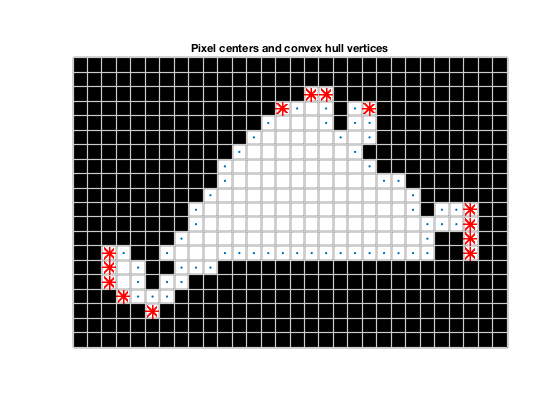Notice that there are some chains of three or more colinear convex hull vertices.

xlim([21.5 32.5])
ylim([9.5 15.5])
title('Colinear convex hull vertices')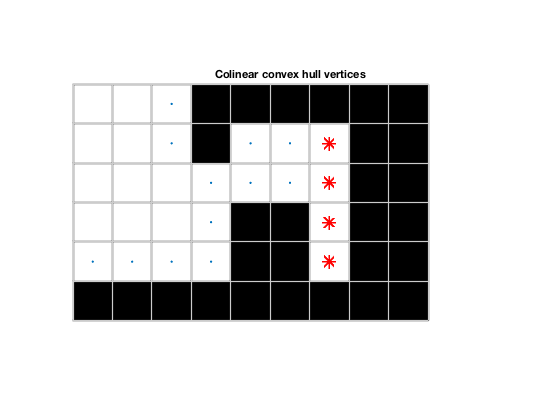In some of the other processing steps related to Feret diameter measurements, colinear convex hull vertices can cause problems. We can eliminate these vertices directly in the call to convhull using the 'Simplify' parameter.

h = convhull(x,y,'Simplify',true);
x_hull = x(h);
y_hull = y(h);
delete(hull_line);
hold on
plot(x_hull,y_hull,'r*','MarkerSize',12)
hold off
title('Colinear hull vertices removed')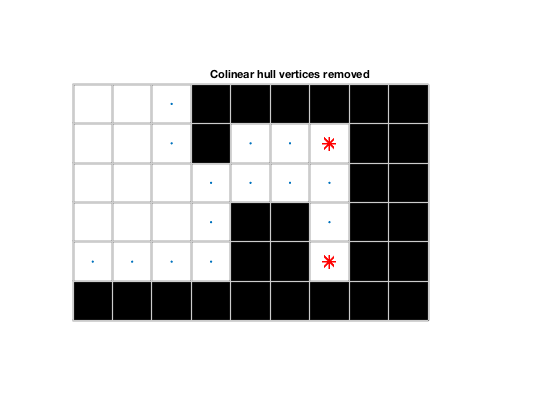imshow(bw)
hold on
plot(x_hull,y_hull,'r-*','LineWidth',2,'MarkerSize',12)
hold off
title('A Blob''s Convex Hull and Its Vertices')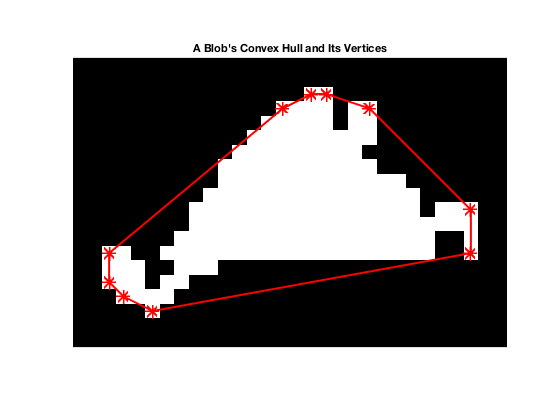Notice, though, that there are white bits showing outside the red convex hull polygon. That's because we are only using the pixel centers.

#### Weaknesses of Using the Pixel Centers

Consider a simpler binary object, one that has only one row.

bw2 = false(5,15);
bw2(3,5:10) = true;
imshow(bw2)
pixelgrid
[y,x] = find(bw2);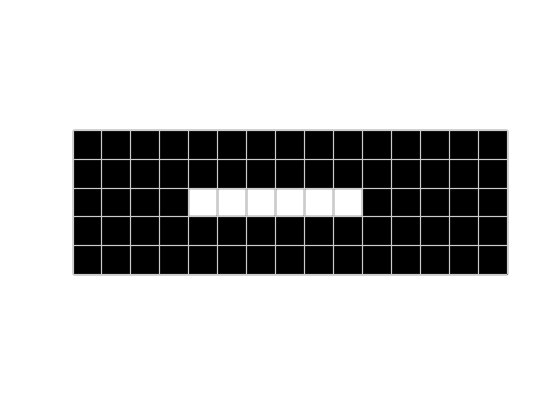The function convhull doesn't even work on colinear points.

try
hull = convhull(x,y,'Simplify',true);
catch e
fprintf('Error message from convhull: "%s"\n', e.message);
end

Error message from convhull: "Error computing the convex hull. The points may be collinear."


But even if it did return an answer, the answer would be a degenerate polygon with length 5 (even though the number of foreground pixels is 6) and zero area.

hold on
plot(x,y,'r-*','MarkerSize',12,'LineWidth',2)
hold off
title('Degenerate convex hull polygon')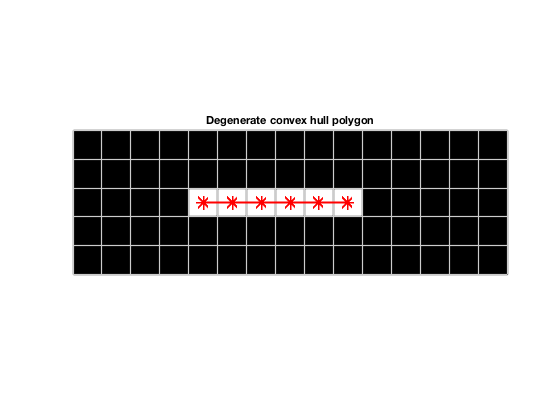We can solve this degeneracy problem by using square pixels.

#### Square Pixels

In the computation of the convex hull above, we treated each pixel as a point. We can, instead, treat each pixel as a square by computing the convex hull of all the corners of every pixel. Here's one way to perform that computation.

offsets = [ ...
0.5  -0.5
0.5   0.5
-0.5  -0.5
-0.5   0.5 ]';

offsets = reshape(offsets,1,2,[]);

P = [x y];
Q = P + offsets;
R = permute(Q,[1 3 2]);
S = reshape(R,[],2);

h = convhull(S,'Simplify',true);
x_hull = S(h,1);
y_hull = S(h,2);

imshow(bw2)
pixelgrid
hold on
plot(x_hull,y_hull,'r-*','MarkerSize',12,'LineWidth',2)
hold off
title('Convex hull of square pixels')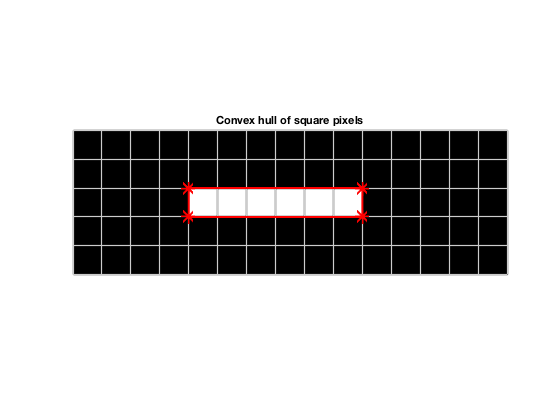This result looks good at first glance. However, it loses some of its appeal when you consider the implications for computing the maximum Feret diameter.

points = [x_hull y_hull];
[d,end_points] = maxFeretDiameter(points,antipodalPairs(points))
hold on
plot(end_points(:,1),end_points(:,2),'k','LineWidth',3)
hold off
title('The maximum Feret diameter is not horizontal')

d =

6.0828

end_points =

10.5000    2.5000
4.5000    3.5000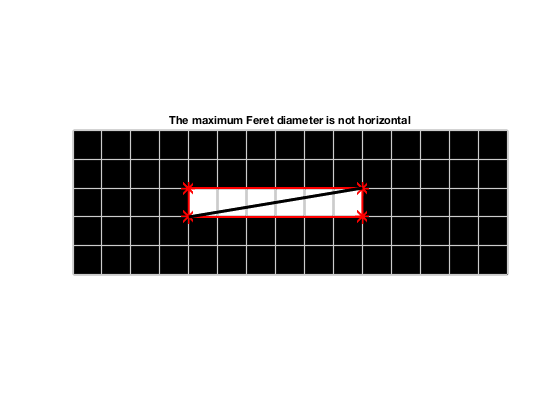The maximum Feret distance of this horizontal segment of is 6.0828 ($\sqrt{37}$) instead of 6, and the corresponding orientation in degrees is:

atan2d(1,6)

ans =

9.4623



Another worthy attempt is to use diamond pixels.

#### Diamond Pixels

Instead of using the four corners of each pixel, let's try using the middle of each pixel edge. Once we define the offsets, the code is exactly the same as for square pixels.

offsets = [ ...
0.5   0.0
0.0   0.5
-0.5   0.0
0.0  -0.5 ]';

offsets = reshape(offsets,1,2,[]);

P = [x y];
Q = P + offsets;
R = permute(Q,[1 3 2]);
S = reshape(R,[],2);

h = convhull(S,'Simplify',true);
x_hull = S(h,1);
y_hull = S(h,2);

imshow(bw2)
pixelgrid
hold on
plot(x_hull,y_hull,'r-*','MarkerSize',12,'LineWidth',2)
hold off
title('Convex hull of diamond pixels')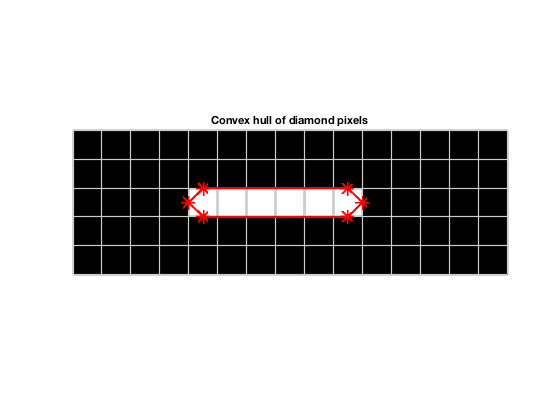Now the max Feret diameter result looks better for the horizontal row of pixels.

points = [x_hull y_hull];
[d,end_points] = maxFeretDiameter(points,antipodalPairs(points))
hold on
plot(end_points(:,1),end_points(:,2),'k','LineWidth',3)
hold off

d =

6

end_points =

10.5000    3.0000
4.5000    3.0000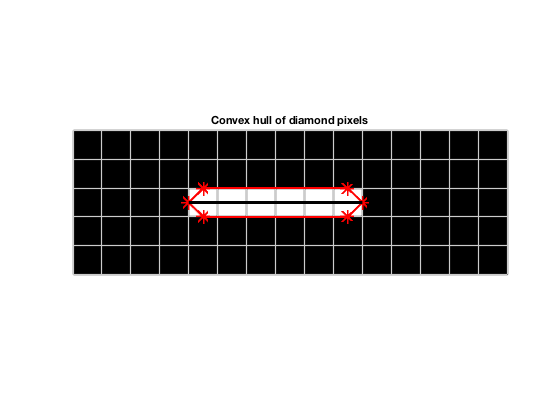Hold on, though. Consider a square blob.

bw3 = false(9,9);
bw3(3:7,3:7) = true;
imshow(bw3)
pixelgrid
[y,x] = find(bw3);
P = [x y];
Q = P + offsets;
R = permute(Q,[1 3 2]);
S = reshape(R,[],2);

h = convhull(S,'Simplify',true);
x_hull = S(h,1);
y_hull = S(h,2);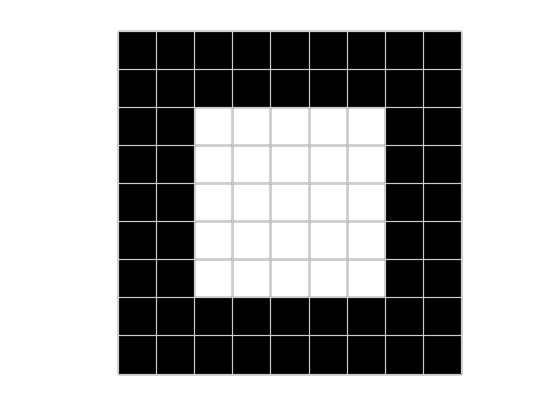hold on
plot(x_hull,y_hull,'r-*','MarkerSize',12,'LineWidth',2)
points = [x_hull y_hull];
[d,end_points] = maxFeretDiameter(points,antipodalPairs(points))
plot(end_points(:,1),end_points(:,2),'k','LineWidth',3)
hold off
title('The max Feret diameter is not at 45 degrees')

d =

6.4031

end_points =

7.5000    3.0000
2.5000    7.0000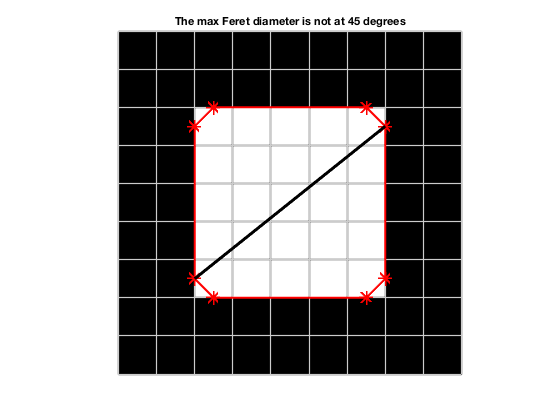We'd like to see the max Feret diameter oriented at 45 degrees, and clearly we don't.

#### Circular Pixels

OK, I'm going to make one more attempt. I'm going to treat each pixel as approximately a circle. I'm going to approximate a circle using 24 points that are spaced at 15-degree intervals along the circumference.

thetad = 0:15:345;
offsets = reshape(offsets,1,2,[]);
Q = P + offsets;
R = permute(Q,[1 3 2]);
S = reshape(R,[],2);

h = convhull(S,'Simplify',true);
x_hull = S(h,1);
y_hull = S(h,2);

imshow(bw3)
pixelgrid
hold on
plot(x_hull,y_hull,'r-*','MarkerSize',12,'LineWidth',2)
points = [x_hull y_hull];
[d,end_points] = maxFeretDiameter(points,antipodalPairs(points))
plot(end_points(:,1),end_points(:,2),'k','LineWidth',3)
axis on
hold off

d =

6.6569

end_points =

7.3536    7.3536
2.6464    2.6464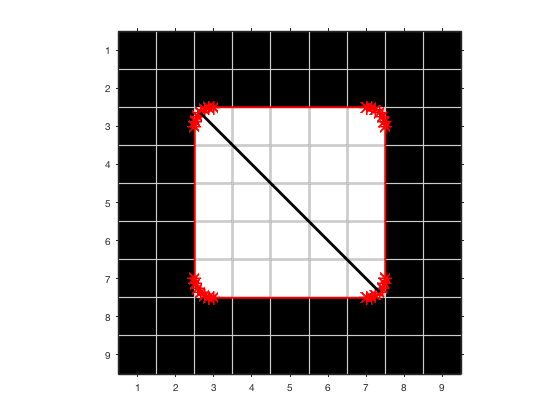Now the max Feret diameter orientation is what we would naturally expect, which is $\pm 45^{\circ}$. The orientation would also be as expected for a horizontal or vertical segment of pixels.

Still, a circular approximation might not always give exactly what a user might expect. Let's go back to the Martha's Vinyard blob that I started with. I wrote a function called pixelHull that can compute the convex hull of binary image pixels in a variety of different ways. The call pixelHull(bw,24) computes the pixel hull using a 24-point circle approximation.

Here's the maximum Feret diameter using that approximation.

imshow(bw)
V = pixelHull(bw,24);
hold on
plot(V(:,1),V(:,2),'r-','LineWidth',2,'MarkerSize',12)
[d,end_points] = maxFeretDiameter(V,antipodalPairs(V));
plot(end_points(:,1),end_points(:,2),'m','LineWidth',3)
axis on
pixelgrid
hold off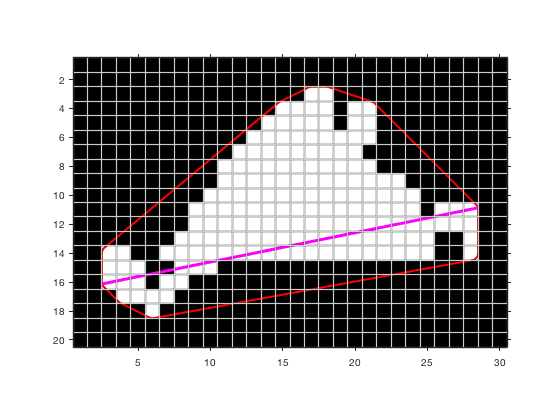I think many people might expect the maximum Feret diameter to go corner-to-corner in this case, but it doesn't exactly do that.

xlim([22.07 31.92])
ylim([8.63 15.20])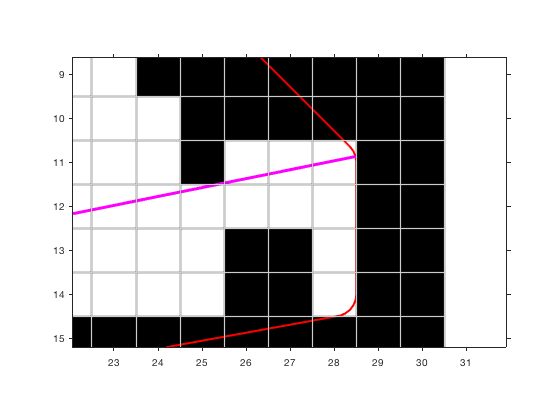You have to use square pixels to get corner-to-corner.

imshow(bw)
V = pixelHull(bw,'square');
hold on
plot(V(:,1),V(:,2),'r-','LineWidth',2,'MarkerSize',12)
[d,end_points] = maxFeretDiameter(V,antipodalPairs(V));
plot(end_points(:,1),end_points(:,2),'m','LineWidth',3)
axis on
pixelgrid
hold off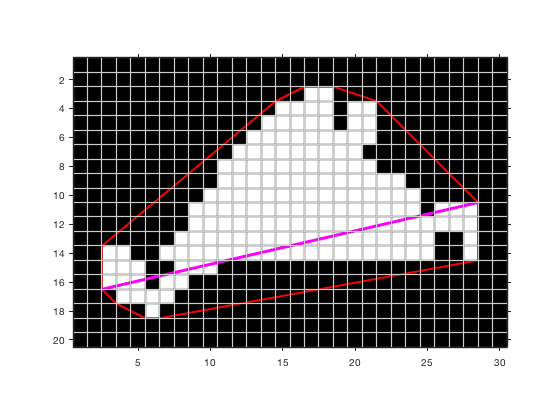xlim([22.07 31.92])
ylim([8.63 15.20])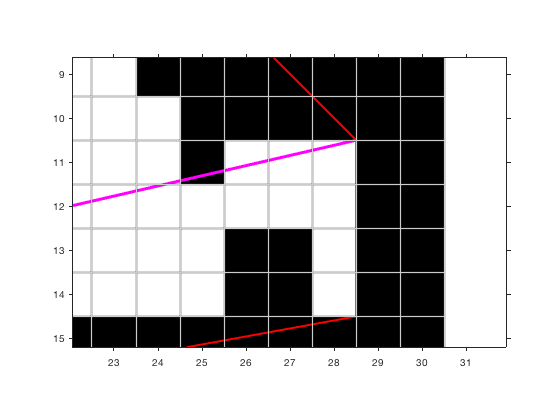After all this, I'm still not completely certain which shape assumption will generally work best. My only firm conclusion is that the point approximation is the worst choice. The degeneracies associated with point pixels are just too troublesome.

If you have an opinion, please share it in the comments. (Note: A comment that says, "Steve, you're totally overthinking this" would be totally legit.)

The rest of the post contains functions used by the code above.

function V = pixelHull(P,type)

if nargin < 2
type = 24;
end

if islogical(P)
P = bwperim(P);
[i,j] = find(P);
P = [j i];
end

if strcmp(type,'square')
offsets = [ ...
0.5  -0.5
0.5   0.5
-0.5   0.5
-0.5  -0.5 ];

elseif strcmp(type,'diamond')
offsets = [ ...
0.5  0
0    0.5
-0.5  0
0   -0.5 ];

else
% type is number of angles for sampling a circle of diameter 1.

end

offsets = offsets';
offsets = reshape(offsets,1,2,[]);

Q = P + offsets;
R = permute(Q,[1 3 2]);
S = reshape(R,[],2);

k = convhull(S,'Simplify',true);
V = S(k,:);
end


Published with MATLAB® R2017b

|